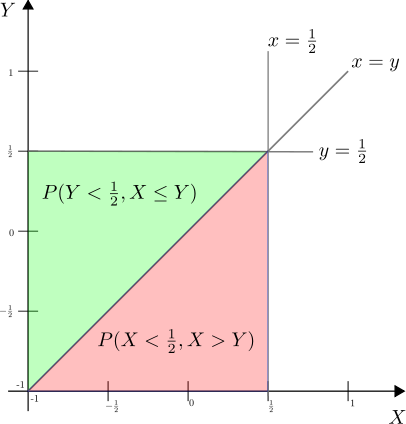# GATE-2012 ECE Q24 (math)Question 24 on math from GATE (Graduate Aptitude Test in Engineering) 2012 Electronics and Communication Engineering paper.

(A) 3/4

(B) 9/16

(C) 1/4

(D) 2/3

## Solution

Let

.

Expanding,The above equation can be represented as shown in the figure below and the total probability is the sum of green and red regions.

The sum of red and green regions is

.

Since and are independent,

.

From probability density function of uniform random variable,

.

Summarizing, the total probability is

.

### Matlab example

clear all;
N    = 10^5;
x    = 2*rand(1,N)-1; % uniform distributed [-1,1]
y    = 2*rand(1,N)-1; % uniform distributed [-1,1]
prob = size(find(max(x,y)<1/2),2)/N

## References

 GATE Examination Question Papers [Previous Years] from Indian Institute of Technology, Madras http://gate.iitm.ac.in/gateqps/2012/ec.pdf

 Wiki entry on Uniform random variables

http://en.wikipedia.org/wiki/Uniform_distribution_(continuous)

 Function of two random variables, notes from Caltech

http://ee162.caltech.edu/notes/lect8.pdf